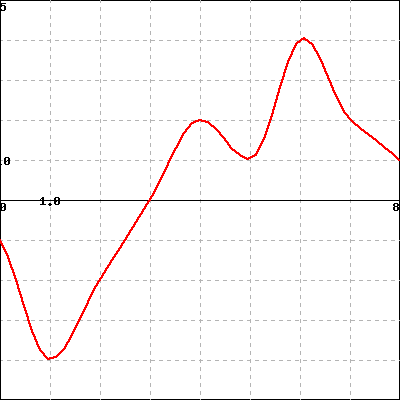Below is the graph of the derivative $f'(x)$ of a function defined on the interval (0,8). You can click on the graph to see a larger version in a separate window.Refer to the graph to answer each of the following questions. For parts (A) and (B), use interval notation to report your answer. (If needed, you use U for the union symbol.)

(A) For what values of $x$ in (0,8) is $f(x)$ increasing? (If the function is not increasing anywhere, enter None .)

(B) For what values of $x$ in (0,8) is $f(x)$ concave down? (If the function is not concave down anywhere, enter None .)
(C) Find all values of $x$ in (0,8) is where $f(x)$ has a local minimum, and list them (separated by commas) in the box below. (If there are no local minima, enter None .)
(D) Find all values of $x$ in (0,8) is where $f(x)$ has an inflection point, and list them (separated by commas) in the box below. (If there are no inflection points, enter None .)